# NCERT solutions for Class 10 Maths: Chapter 13 Surface Area and Volume Exercise 13.2

In this page we have NCERT solutions for Class 10 Maths: Chapter 13 Surface Area and Volume for Exercise 13.2 on pages 247 and 248. Hope you like them and do not forget to like , social_share and comment at the end of the page.

This exercise is based on the volume of an object formed by combining any two of the basic solids, namely, cuboid, cone, cylinder, sphere and hemisphere
Formula to used
The total Volume of the any new solid is the sum of the Volume of each of the individual parts.
Volume of new solid = Volume of One part + Volume of Second part + Volume of Third part
Volume of Common Shapes
Volume of cone
$=\frac {1}{3}\pi r^2h$
Volume of Sphere
$=\frac {4}{3} \pi r^3$
Volume of Cylinder
$=\pi r^2 h$
Unless stated otherwise, take $\pi = \frac {22}{7}$.

## Surface Area and Volume Exercise 13.2

Question 1
A solid is in the shape of a cone standing on a hemisphere with both their radii being equal to 1 cm and the height of the cone is equal to its radius. Find the volume of the solid in terms of $\pi$.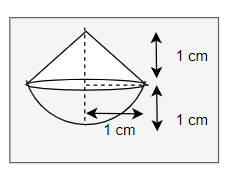Given
height = 1 cm
Volume of hemisphere
$=\frac {2}{3} \pi r^3$
$= \frac {2}{3} \pi$ cm3
Volume of cone
$=\frac {1}{3}\pi r^2h$
$= \frac {1}{3} \pi$ cm3
Total volume
$= \frac {2}{3} \pi + \frac {1}{3} \pi$
= π cm3

Question 2
Rachel, an engineering student, was asked to make a model shaped like a cylinder with two cones attached at its two ends by using a thin aluminium sheet. The diameter of the model is 3 cm and its length is 12 cm. If each cone has a height of 2 cm, find the volume of air contained in the model that Rachel made. (Assume the outer and inner dimensions of the model to be nearly the same.)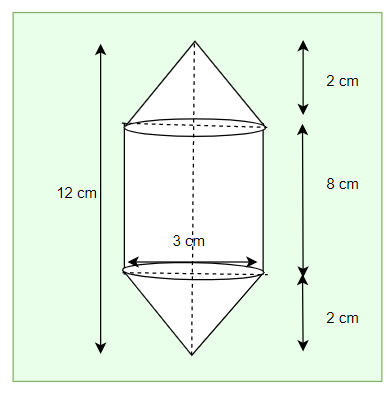Height of cylinder = 12 – 4 = 8 cm
Height of cone = 2 cm
Volume of cylinder = πr2h = 18 π cm3
Volume of cone
$=\frac {1}{3}\pi r^2h$
=1.5 π cm3
Total volume
= Volume of cylinder + 2 × (Volume of cone)
=66 cm3

Question 3
A gulab jamun, contains sugar syrup up to about 30% of its volume. Find approximately how much syrup would be found in 45 gulab jamuns, each shaped like a cylinder with two hemispherical ends with length 5 cm and diameter 2.8 cm.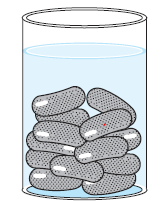Length of cylinder = 5 – 2.8 = 2.2 cm, radius = 1.4 cm
Volume of cylinder= πr2h = 4.312 π cm3
Volume of two hemispheres
$=\frac {4}{3} \pi r^3$
= (10.976/3) π cm3
Total volume of One Gulab Jamun
= Volume of Cylinder + Volume of two hemispheres
=25.05 cm3
Volume of syrup = 30% of total volume
=30% (25.05 cm3)
=7.515 cm3
Volume of syrup in 45 gulab jamun = 45 x 7.515 = 338.184 cm3

Question 4
A pen stand made of wood is in the shape of a cuboid with four conical depressions to hold pens. The dimensions of the cuboid are 15 cm by 10 cm by 3.5 cm. The radius of each of the depressions is 0.5 cm and the depth is 1.4 cm. Find the volume of wood in the entire stand.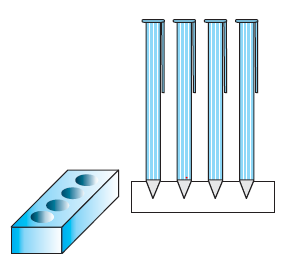Dimensions of cuboid = 15 cm x 10 cm x 3.5 cm, radius of cone = 0.5 cm, depth of cone = 1.4 cm
Volume of cuboid = length x width x height
= 15 x 10 x 3.5 = 525 cm3
Volume of cone
$=\frac {1}{3}\pi r^2h$
=11/30 cm3
Volume of wood = Volume of cuboid – 6 x volume of cone
= 525 – 6 × (11/30)
=522.8 cm3

Question 5
A vessel is in the form of an inverted cone. Its height is 8 cm and the radius of its top, which is open, is 5 cm. It is filled with water up to the brim. When lead shots, each of which is a sphere of radius 0.5 cm are dropped into the vessel, one-fourth of the water flows out. Find the number of lead shots dropped in the vessel.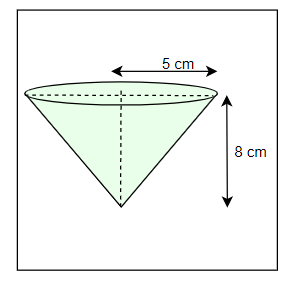Given Radius of cone = 5 cm, height of cone = 8 cm, radius of sphere = 0.5 cm
Volume of cone
$=\frac {1}{3}\pi r^2h$
= (200/3) π cm3
So Volume of water overflown= (1/4)× (200/3) π =(50/3) π
$=\frac {4}{3} \pi r^3$
= (1/6) π
= Volume of Water overflown/ Volume of Lead shot
= (50/3) π /(1/6) π
=100
Question 6
A solid iron pole consists of a cylinder of height 220 cm and base diameter 24 cm, which is surmounted by another cylinder of height 60 cm and radius 8 cm. Find the mass of the pole, given that 1 cm3 of iron has approximately 8g mass.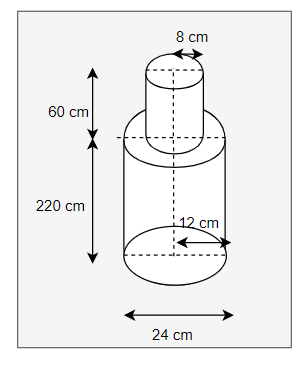Radius of bigger cylinder = 12 cm, height of bigger cylinder = 220 cm
Radius of smaller cylinder = 8 cm, height of smaller cylinder = 60 cm
Volume of bigger cylinder
= πr2h
=(22/7)×(12)2×220
=99565.8 cm3
Volume of Smaller cylinder
= πr2h
= (22/7)×(8)2×60
=12068.5 cm3
Total volume
=99565.8 cm3 + 12068.5 cm3
=111634.5 cm3
Mass = Density x volume
=8×111634.5
=893 Kg

Question 7
A solid consisting of a right circular cone of height 120 cm and radius 60 cm standing on a hemisphere of radius 60 cm is placed upright in a right circular cylinder full of water such that it touches the bottom. Find the volume of water left in the cylinder, if the radius of the cylinder is 60 cm and its height is 180 cm.
Given
Radius of cone = 60 cm, height of cone = 120 cm
Radius of hemisphere = 60 cm
Radius of cylinder = 60 cm, height of cylinder = 180 cm
Volume of cone
$=\frac {1}{3}\pi r^2h$
=144×103 π cm3
Volume of hemisphere
=(2/3) πr3
=144×103 π cm3
Volume of solid
= Volume of Cone + Volume of hemisphere
=144×103 π cm3 + 144×103 π cm3
=288×103 π cm3
Volume of cylinder
=πr2h
=648×103 π cm3
Volume of water left in the cylinder
=Volume of Cylinder – Volume of Solid
=103 π ( 648-288)
=1130400 cm3

Question 8
A spherical glass vessel has a cylindrical neck 8 cm long, 2 cm in diameter; the diameter of the spherical part is 8.5 cm. By measuring the amount of water, it holds, a child finds its volume to be 345 cm3. Check whether she is correct, taking the above as the inside measurements, and π = 3.14.
Solution:
Given as per Question
Radius of cylinder = 1 cm
Height of cylinder = 8 cm
Radius of sphere = 8.5 cm
Volume of cylinder
=πr2h
=8π cm3
=25.12 cm3
Volume of sphere
$=\frac {4}{3}\pi r^3$
= 321.4 cm3
Total volume
=321.4 cm3 + 25.12 cm3
=346.51 cm3

## Summary

1. Class 10 Maths NCERT solutions for Surface Area and Volume Exercise 13.2 has been prepared by Expert with utmost care. If you find any mistake.Please do provide feedback on mail.You can download this as pdf
2. This chapter 13 has total 5 Exercise 13.1 ,13.2,13.3 ,13.4 and 13.5. This is the Second exercise in the chapter.You can explore previous exercise of this chapter by clicking the link below

• Notes
• Assignments
• NCERT Solutions
• NCERT Solution Surface Area and Volume Class10 Exercise 13.1
• NCERT Solution Surface Area and Volume Class10 Exercise 13.2
• NCERT Solution Surface Area and Volume Class10 Exercise 13.3Go back to Class 10 Main Page using below links

### Practice Question

Question 1 What is $1 - \sqrt {3}$ ?
A) Non terminating repeating
B) Non terminating non repeating
C) Terminating
D) None of the above
Question 2 The volume of the largest right circular cone that can be cut out from a cube of edge 4.2 cm is?
A) 19.4 cm3
B) 12 cm3
C) 78.6 cm3
D) 58.2 cm3
Question 3 The sum of the first three terms of an AP is 33. If the product of the first and the third term exceeds the second term by 29, the AP is ?
A) 2 ,21,11
B) 1,10,19
C) -1 ,8,17
D) 2 ,11,20